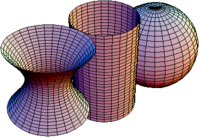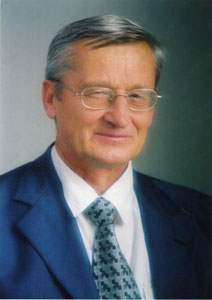Alexander Andreevich Borisenko was born in May 24, 1946 in Lebedin town (Sumy reg, Ukraine). In 1969 he graduated from the Mechanics and Mathematics Department of Kharkiv State University and enrolled in the geometry graduate school. In 1973 he defended his PhD thesis, “The structure of surfaces with degenerate spherical image” (Kharkiv State University), and in 1983 he defended his habilitation thesis “Multi-dimensional surfaces of nonpositive extrinsic curvature” (Moscow State University). In 1995 he was elected as a corresponding member of the NAS of Ukraine. In 2002 A. Borisenko was awarded the Krylov Prize of the National Academy of Sciences of Ukraine, followed by the State Prize of Ukraine in the field of science and technology in 2005 and A.V.Pogorelov Prize of National Academy of Sciences of Ukraine in 2010.
Since 1973 he worked at Kharkiv State University in the department of geometry, and from 1980 to 2012 headed the department of geometry. During this period the Kharkiv geometrical school got a new impulse in development of the geometry of multidimensional submanifolds in Euclidean, Riemannian, pseudo-Riemannian, Finsler spaces and spaces with additional structures (complex, Sasakian, fiber, etc.). His research interests are very much “multivariate”. To prove this it is enough to look at the list his different years post-graduate students and collaborators: D. Bolotov (geometry and topology of foliations), K. Drach (isoperimetric inequalities, comparison theorem, the optimal control), N. Farafonova (geometry of Grassmann bundles), V. Lisitsa (geometry helical submanifolds), O. Lykova (geometry of the Grassmann image of complex submanifolds), V. Miquel (curvature flows, geometry of convex hypersurfaces in Hadamard manifolds), Yu. Nikolayevsky (geometry of the Grassmann image of submanifolds), S. Okrut (geometry of distributions on manifolds), Ye. Olin (Finsler geometry), E. Petrov (geometry of submanifolds Lie groups), L. Sergienko (nonholonomic geometry), K. Tenenblat (Minkowski geometry), V. Ushakov (geometry of ruled submanifolds), A. Yampolsky (geometry of fiber bundles). Since 2012 is a professor of the department of mathematical analysis and optimization methods of Sumy State University. Since 2012 Alexander Andreevich is a professor of the department of mathematical analysis and optimization methods of Sumy State University.
As a visiting professor, he worked in the University of Haifa (Israel, 2013, 2010), Technical University of Berlin (Germany, 2010), University of Rome “LaSapienza” (Italy, 2003), Autonomous University of Barcelona (Spain, 2000), Centre de Recerca Mathematica (Barcelona, 2000), University of Valencia (Spain, 1997, 1999, 2000, 2004, 2008), University of Brasilia (Brazil, 1996, 2008), Bilkent University (Turkey, 1995). A. Borisenko took part as a Speaker in the International Congress of Mathematicians in Beijing (2002) and Zurich (1994).
Alexander Andreevich is the author of more than 120 articles and two monographs (Intrinsic and extrinsic geometry of multidimensional manifolds / A. Borisenko - M.: Examen, 2003. - 670 p.; An introduction to Hamilton and Perelman's work on the conjectures of Poincare and Thurston / A. Borisenko, E. Cabezas-Rivas, V. Miquel-Molina. - Matematiques, 2006. - 3 (2) - 150 p.). He is the author of textbooks in Analytical Geometry (1993) and Differential Geometry and Topology (1995) for undergraduates.